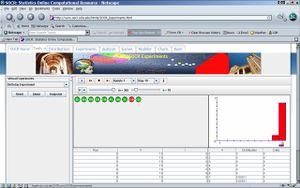# SOCR EduMaterials Activities Birthday

## SOCR Educational Materials - Activities - SOCR Birthday Experiment Assignment

Assignment Goals: To understand the computation of the probability that at least two people in a group of m people share the same birthday and the probability that nobody in a group of m people share the same birthday. This activity is the second part of the complete Birthday problem materials.

URL: To understand the idea of those probabilities try the Birthday Experiment applet at the following website: http://socr.stat.ucla.edu/htmls/SOCR_Experiments.html. Select the Birthday Experiment from the drop-down list on the top-left.• Exercise 1: Draw 10 people at random from the population and observe their birthdays. This is like drawing 10 balls (n=10) at random, with replacement, from an urn containing 365 balls (m=365). To do that, set n=1, m=365 and set update=1 and stop=1. Click on the <RUN> button or the one-step button <STEP>.
• How many distinct balls did you get? __________________
• Is that number the same as the value of V?
• What is the value of V ? ____________
• Were there any two balls that repeated? (i.e., any red ball in your sample set?) __________. \(I\) should be 1, if there are two balls with the same number, (the number in the green ball). If not, \(I\) should be 0. What is your I? ________________
• Exercise 2: Above we did only one run of the experiment. That won’t take us too far. We need to repeat the same experiment many times to see how often we get 10 distinct (green) balls and how often we get at least one red ball. Keep n=10 and m=365 and set update=1 and stop=10. You will only see that last run of the experiment in the coins drawn, but you will see all 10 runs and the V’s and I’s and the distribution of the I’s.
• Was there a red ball in the last run? (The last one is the one shown on the bottom of the screen)___
• In how many runs was the number of distinct balls equal to 10? _________________
• In how many runs was V equal to 10? ____________________
• In how many runs was I equal to 0? ______________________
• In how many runs was I equal to 1? _______________________
• What is the distribution of I on the top-right hand side giving you then? _______________
• According to your 10 runs, the probability that no two people in a group of 10 share the same birthday is? _____________________________
• The probability that at least two people in a group of 10 share the same birthday is, according to your 10 runs _________________

• Exercise 3: With 10 runs, you have not got too close to the true probability. So, what about trying 10,000 runs. Don’t even dream of seeing every single one of these. So, <RESET> the screen, and set the stop at 10,000 and the update at 1,000. This means you will only see the outcome of one in 1,000 runs, even though the computer is generating all 10,000 experiments. But, the final distribution with all 10,000 runs will appear on the right. In blue you’ll se the theoretical distribution and in red you’ll see the sampling distribution. Are these similar?
• Look at the distribution of I on the right. What proportion of times did the run have at least a red ball? __________________. This is the probability that at least two people in a group of 10 share the same birthday. So what is that probability?
• What proportion of the 10,000 experiments had all 10 balls be distinct? _________________This is the probability that nobody in a group of 10 share the same birthday.
• What is then that probability?_____________
• How is the first probability, which you calculated using only 10 experiments above, related to the second one you just did?

• Exercise 4: Use what you have learned above to determine empirically the probability that at least 2 people in a group of 5 share the same birthmonth. Determine also the probability that nobody in a group of 5 share the same month. Write your answer here and attach a printout/screenshot of the applet with the final runs.

• Exercise 5: Use what you have learned above to determine empirically how large should the group of people observed be, for the probabilities of at least two same birthdays and the probability of nobody same birthday to be 50%-50%. This may take some trial and error. Turn in your final answer written here and attach your applet. Use the sampling distribution (in red) and the theoretical exact distribution (in blue).